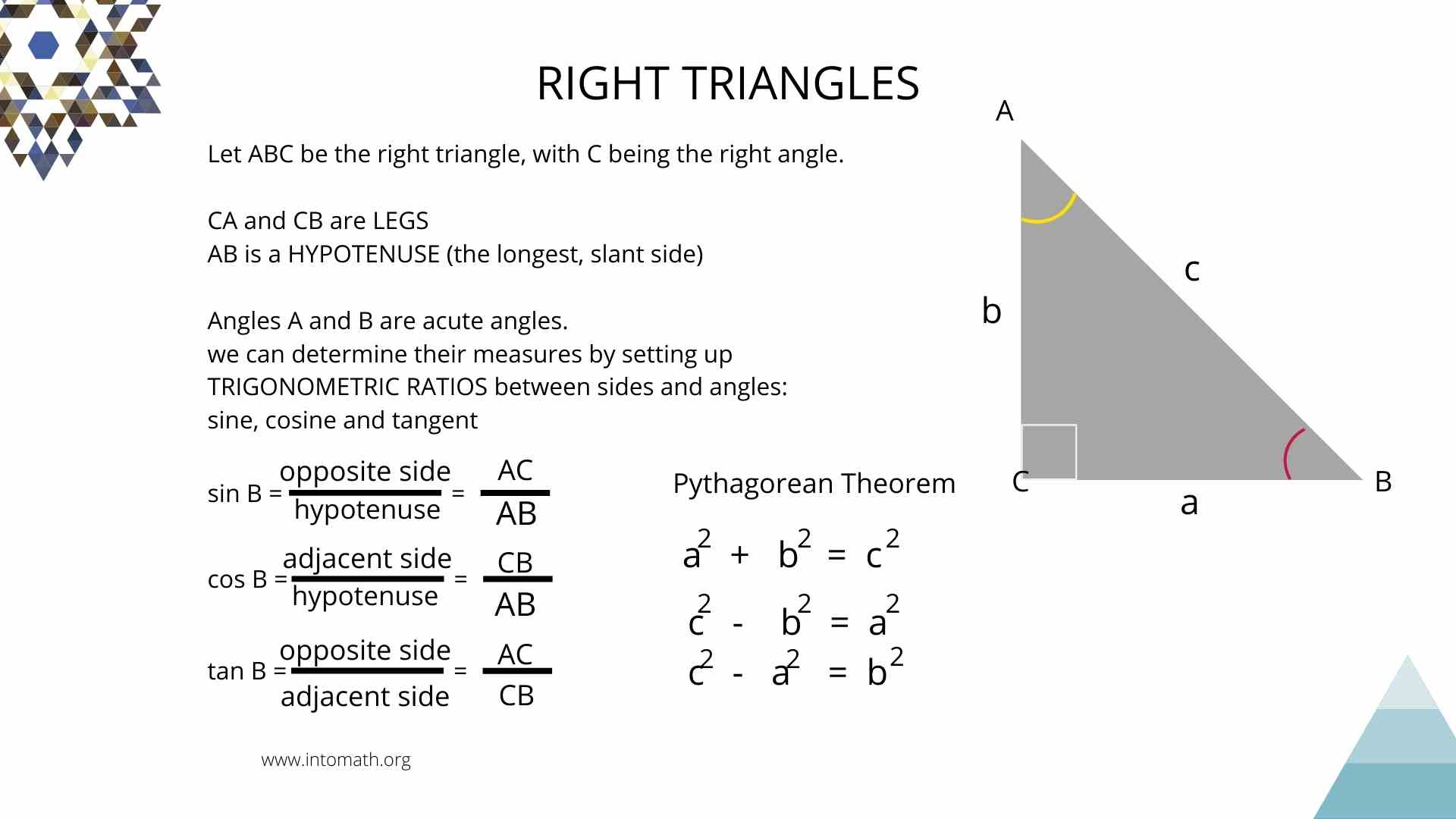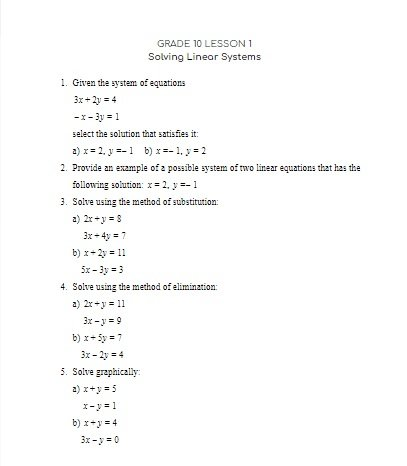CA\$2.00

Right triangle trigonometry is a branch of mathematics that deals with angles and sides in a right triangle.

A right triangle is a triangle with one right angle. The other two angles add up to 90o. Together, the sum of all interior angles in a right triangle is 180o.

The side relationships in a right triangle are based on the Pythagorean Theorem. If the triangle is right, then the following will be true for the three sides of the triangle:

c2 = a2 + b2 where a and b are shorter sides and c is the longest side.

When the lengths of any two sides are known, the third side can always be found using the Pythagorean Theorem.

When two sides are given and a measure of an angle other than the right angle needs to be found. Or only one side and an angle other than the right angle are given – trigonometric ratios should be used.

Trigonometric ratios are ratios between any two sides of a right triangle that can then be used to determine the measure of an angle between those two sides.CA\$4.00

Primary trigonometric ratios (in a right triangle trigonometry) are:

sin(x), cos(x) and tan(x)

sin(x) = opposite/hypotenuse